Скачать презентацию Multivariate Models Analysis of Variance and Regression Using

675141c87da103437962de3b55ba632d.ppt

• Количество слайдов: 23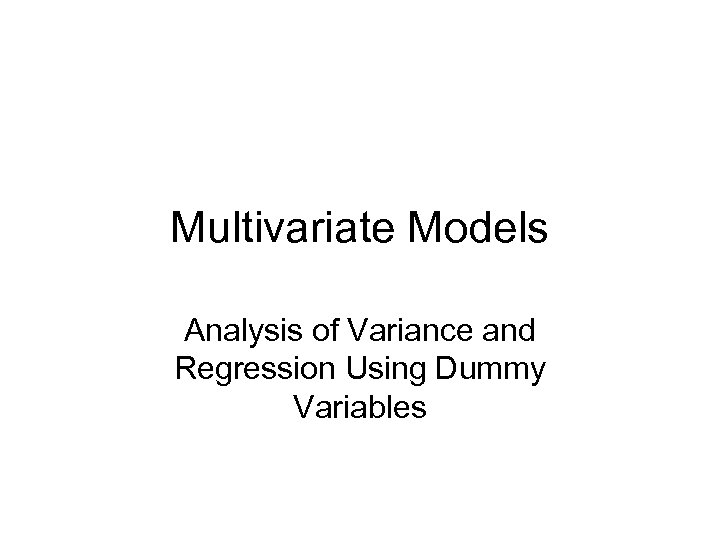Multivariate Models Analysis of Variance and Regression Using Dummy Variables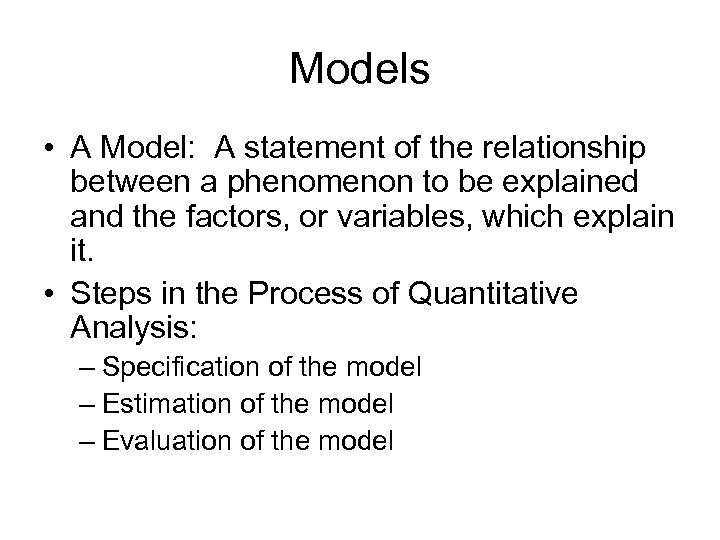Models • A Model: A statement of the relationship between a phenomenon to be explained and the factors, or variables, which explain it. • Steps in the Process of Quantitative Analysis: – Specification of the model – Estimation of the model – Evaluation of the model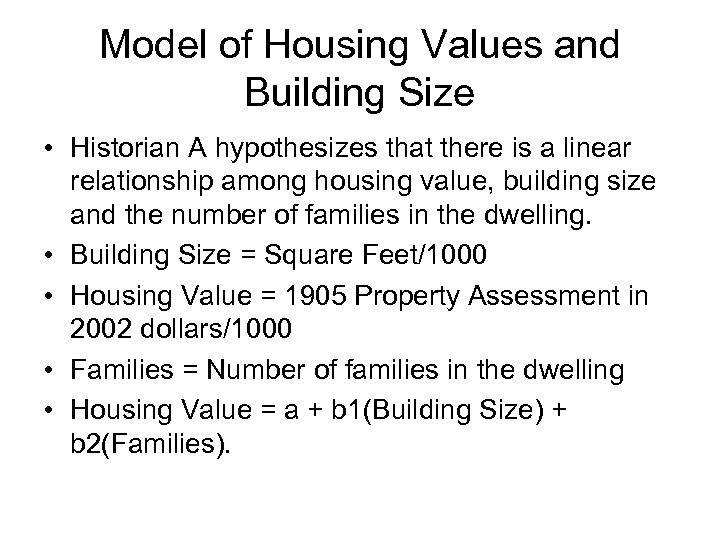Model of Housing Values and Building Size • Historian A hypothesizes that there is a linear relationship among housing value, building size and the number of families in the dwelling. • Building Size = Square Feet/1000 • Housing Value = 1905 Property Assessment in 2002 dollars/1000 • Families = Number of families in the dwelling • Housing Value = a + b 1(Building Size) + b 2(Families).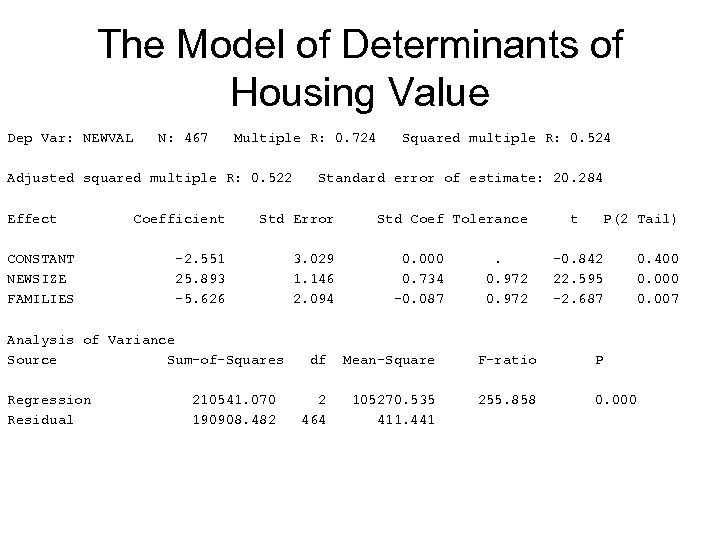The Model of Determinants of Housing Value Dep Var: NEWVAL N: 467 Multiple R: 0. 724 Adjusted squared multiple R: 0. 522 Effect CONSTANT NEWSIZE FAMILIES Regression Residual Standard error of estimate: 20. 284 Coefficient Std Error -2. 551 25. 893 -5. 626 3. 029 1. 146 2. 094 Analysis of Variance Source Sum-of-Squares 210541. 070 190908. 482 Squared multiple R: 0. 524 Std Coef Tolerance 0. 000 0. 734 -0. 087 . 0. 972 t P(2 Tail) -0. 842 22. 595 -2. 687 df Mean-Square F-ratio P 2 464 105270. 535 411. 441 255. 858 0. 000 0. 400 0. 007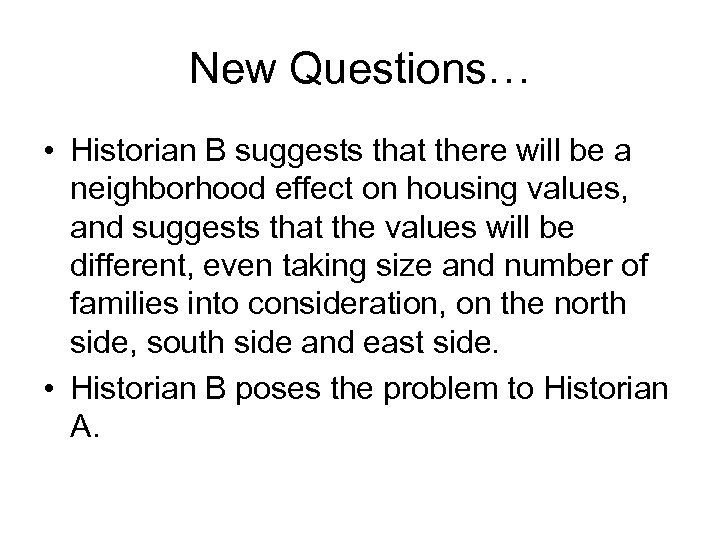New Questions… • Historian B suggests that there will be a neighborhood effect on housing values, and suggests that the values will be different, even taking size and number of families into consideration, on the north side, south side and east side. • Historian B poses the problem to Historian A.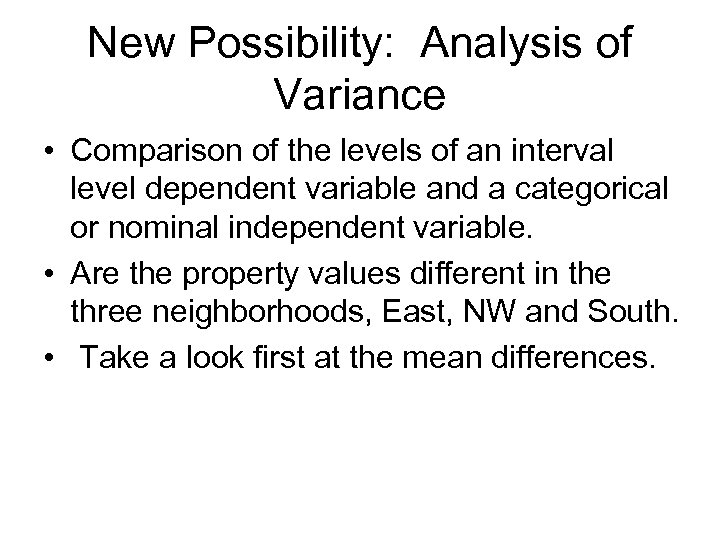New Possibility: Analysis of Variance • Comparison of the levels of an interval level dependent variable and a categorical or nominal independent variable. • Are the property values different in the three neighborhoods, East, NW and South. • Take a look first at the mean differences.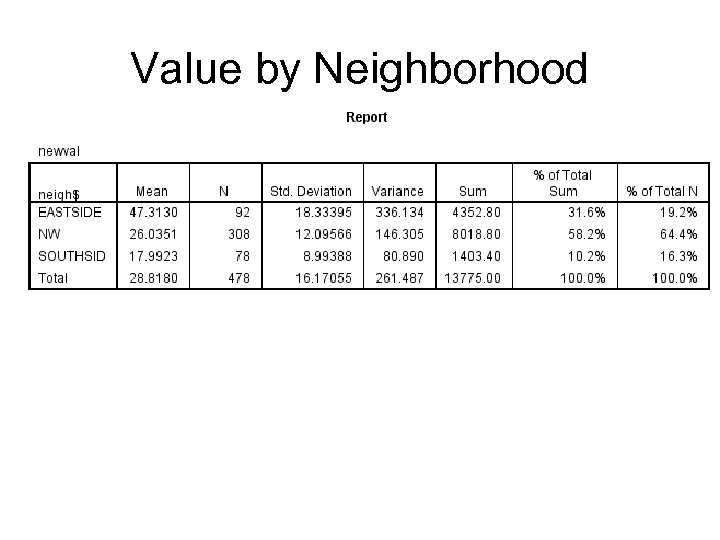Value by Neighborhood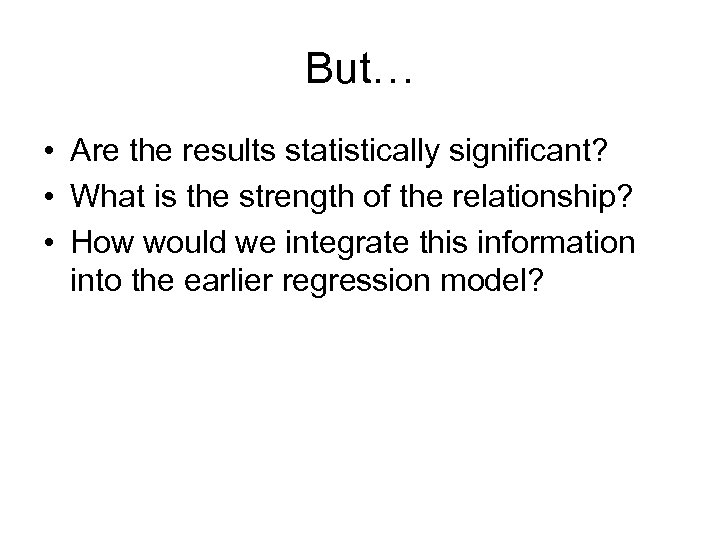But… • Are the results statistically significant? • What is the strength of the relationship? • How would we integrate this information into the earlier regression model?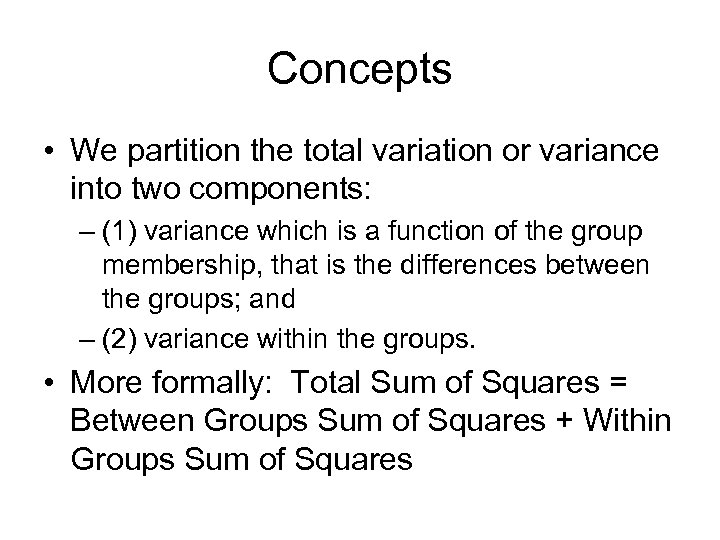Concepts • We partition the total variation or variance into two components: – (1) variance which is a function of the group membership, that is the differences between the groups; and – (2) variance within the groups. • More formally: Total Sum of Squares = Between Groups Sum of Squares + Within Groups Sum of Squares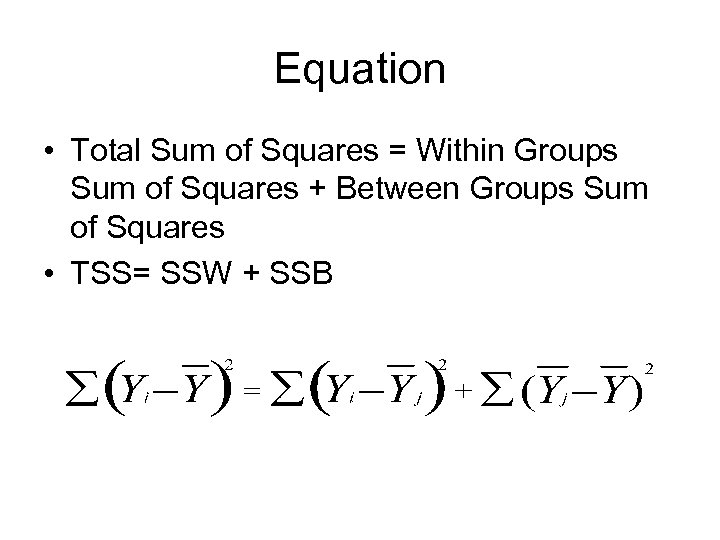Equation • Total Sum of Squares = Within Groups Sum of Squares + Between Groups Sum of Squares • TSS= SSW + SSB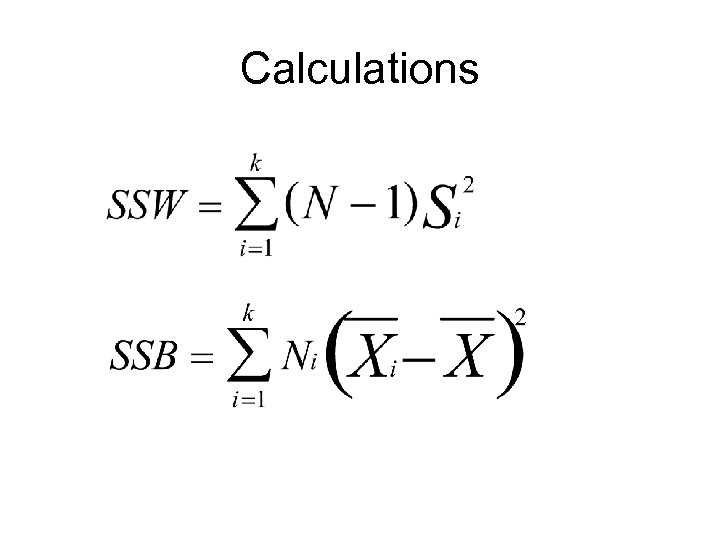Calculations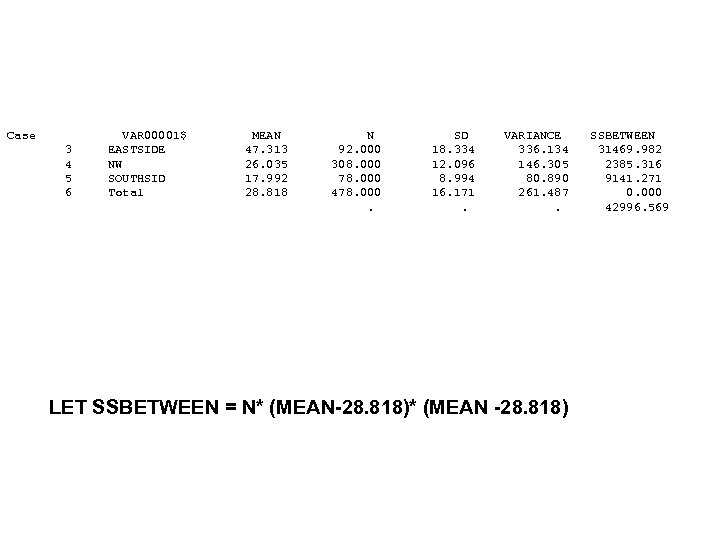Case 3 4 5 6 VAR 00001\$ EASTSIDE NW SOUTHSID Total MEAN 47. 313 26. 035 17. 992 28. 818 N 92. 000 308. 000 78. 000 478. 000. SD 18. 334 12. 096 8. 994 16. 171. VARIANCE 336. 134 146. 305 80. 890 261. 487. LET SSBETWEEN = N* (MEAN-28. 818)* (MEAN -28. 818) SSBETWEEN 31469. 982 2385. 316 9141. 271 0. 000 42996. 569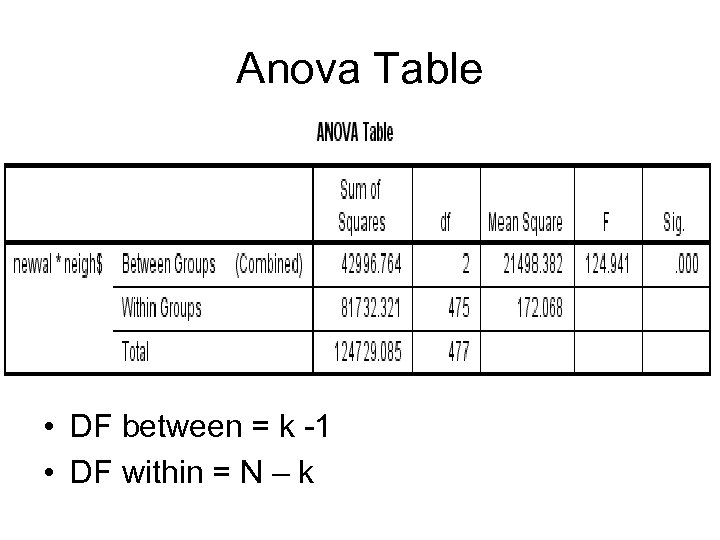Anova Table • DF between = k -1 • DF within = N – k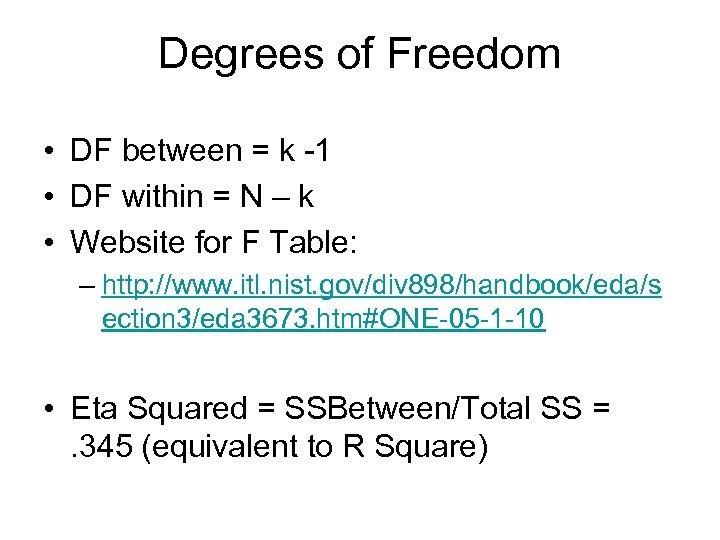Degrees of Freedom • DF between = k -1 • DF within = N – k • Website for F Table: – http: //www. itl. nist. gov/div 898/handbook/eda/s ection 3/eda 3673. htm#ONE-05 -1 -10 • Eta Squared = SSBetween/Total SS =. 345 (equivalent to R Square)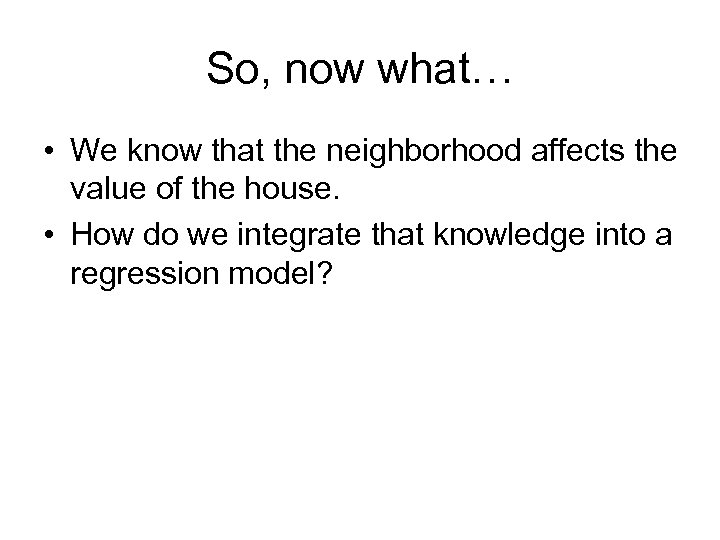So, now what… • We know that the neighborhood affects the value of the house. • How do we integrate that knowledge into a regression model?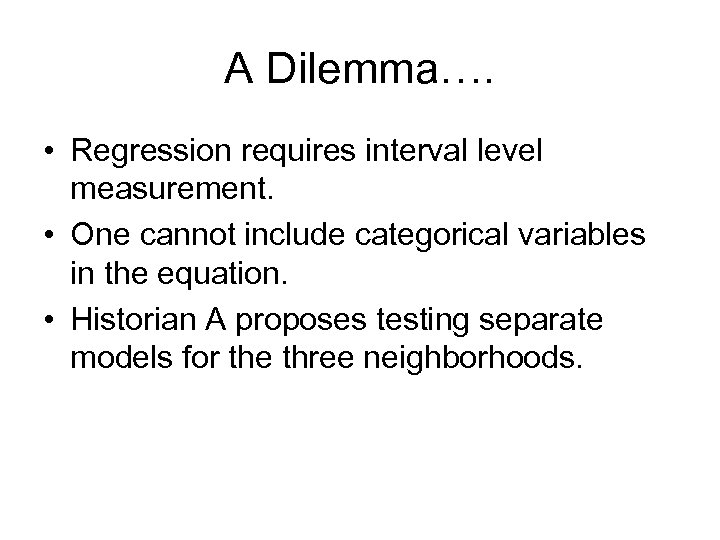A Dilemma…. • Regression requires interval level measurement. • One cannot include categorical variables in the equation. • Historian A proposes testing separate models for the three neighborhoods.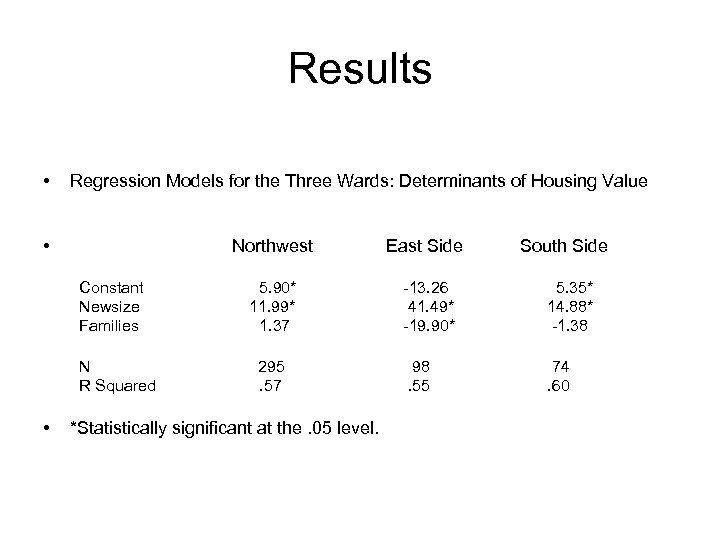Results • Regression Models for the Three Wards: Determinants of Housing Value • Northwest Constant Newsize Families N R Squared • East Side 5. 90* 11. 99* 1. 37 -13. 26 41. 49* -19. 90* 5. 35* 14. 88* -1. 38 98. 55 74. 60 295. 57 *Statistically significant at the. 05 level. South Side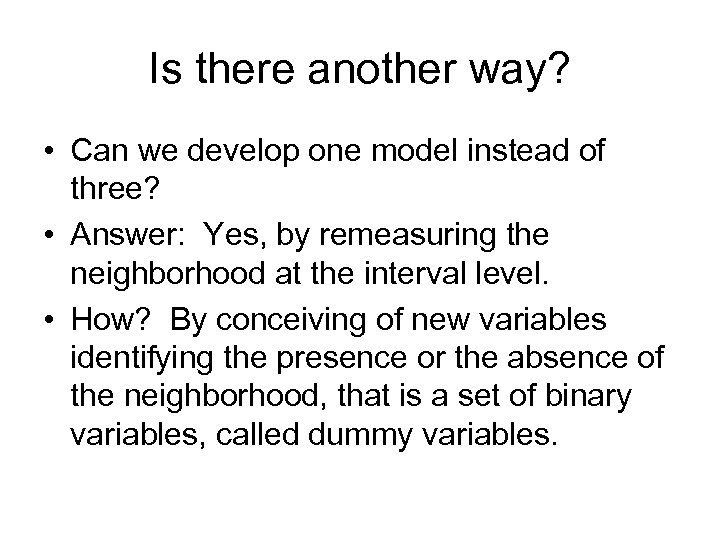Is there another way? • Can we develop one model instead of three? • Answer: Yes, by remeasuring the neighborhood at the interval level. • How? By conceiving of new variables identifying the presence or the absence of the neighborhood, that is a set of binary variables, called dummy variables.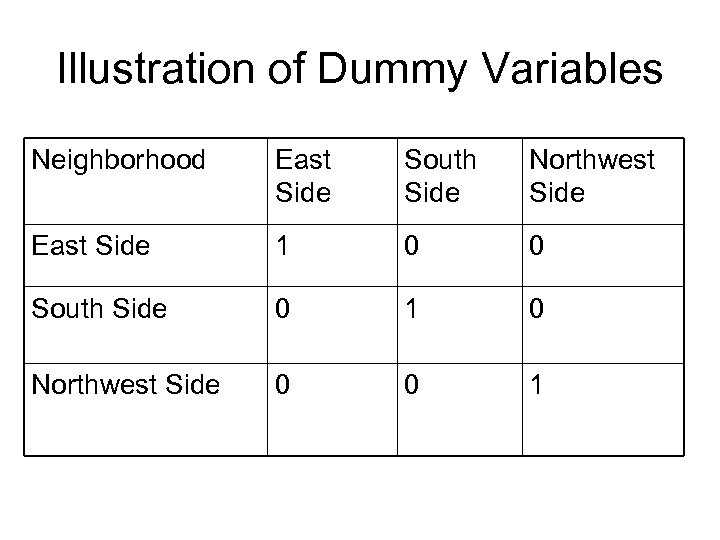Illustration of Dummy Variables Neighborhood East Side South Side Northwest Side East Side 1 0 0 South Side 0 1 0 Northwest Side 0 0 1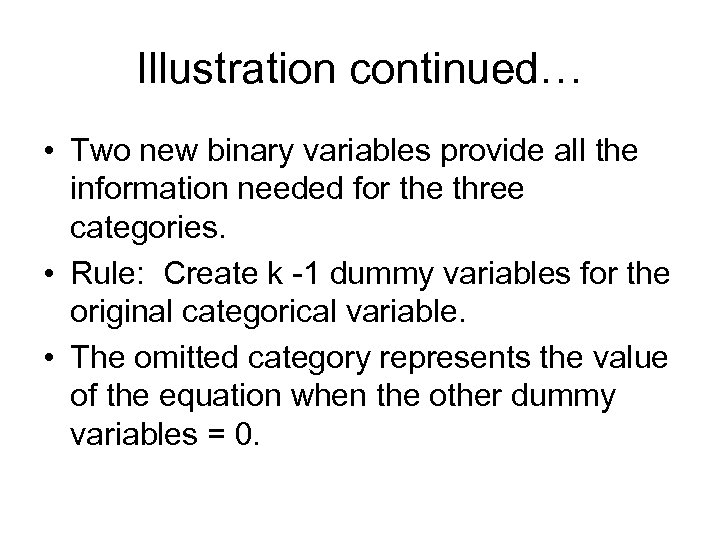Illustration continued… • Two new binary variables provide all the information needed for the three categories. • Rule: Create k -1 dummy variables for the original categorical variable. • The omitted category represents the value of the equation when the other dummy variables = 0.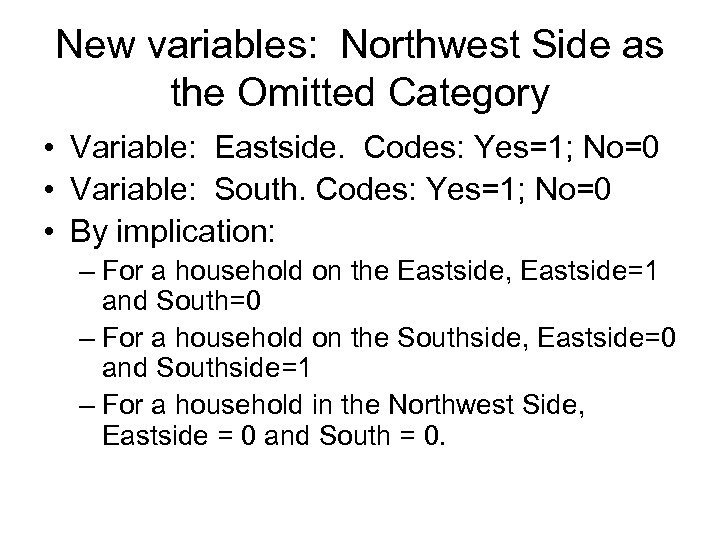New variables: Northwest Side as the Omitted Category • Variable: Eastside. Codes: Yes=1; No=0 • Variable: South. Codes: Yes=1; No=0 • By implication: – For a household on the Eastside, Eastside=1 and South=0 – For a household on the Southside, Eastside=0 and Southside=1 – For a household in the Northwest Side, Eastside = 0 and South = 0.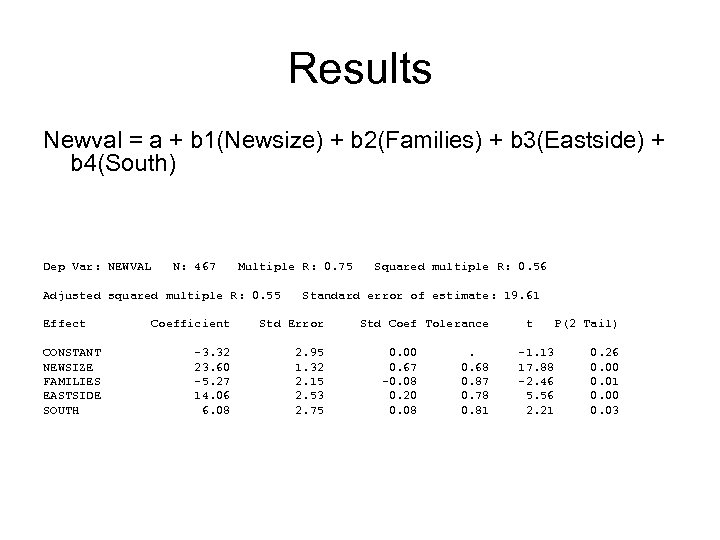Results Newval = a + b 1(Newsize) + b 2(Families) + b 3(Eastside) + b 4(South) Dep Var: NEWVAL N: 467 Multiple R: 0. 75 Adjusted squared multiple R: 0. 55 Effect CONSTANT NEWSIZE FAMILIES EASTSIDE SOUTH Squared multiple R: 0. 56 Standard error of estimate: 19. 61 Coefficient Std Error -3. 32 23. 60 -5. 27 14. 06 6. 08 2. 95 1. 32 2. 15 2. 53 2. 75 Std Coef Tolerance 0. 00 0. 67 -0. 08 0. 20 0. 08 . 0. 68 0. 87 0. 78 0. 81 t -1. 13 17. 88 -2. 46 5. 56 2. 21 P(2 Tail) 0. 26 0. 00 0. 01 0. 00 0. 03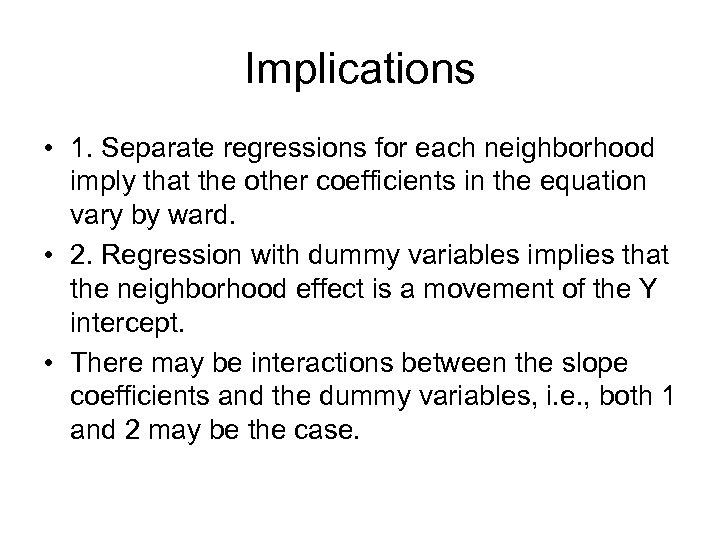Implications • 1. Separate regressions for each neighborhood imply that the other coefficients in the equation vary by ward. • 2. Regression with dummy variables implies that the neighborhood effect is a movement of the Y intercept. • There may be interactions between the slope coefficients and the dummy variables, i. e. , both 1 and 2 may be the case.